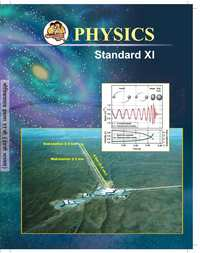# Balbharati solutions for Physics 11th Standard Maharashtra State Board chapter 14 - Semiconductors [Latest edition]

#### Chapters## Chapter 14: Semiconductors

ExercisesExercise
Exercises, Exercise [Page 256]

### Balbharati solutions for Physics 11th Standard Maharashtra State Board Chapter 14 Semiconductors Exercises, Exercise [Page 256]

Exercises | Q 1. (i) | Page 256

Choose the correct option.

Electric conduction through a semiconductor is due to:

• Electrons

• holes

• none of these

• both electrons and holes

Exercises | Q 1. (ii) | Page 256

Choose the correct option.

The energy levels of holes are:

• in the valence band

• in the conduction band

• in the bandgap but close to valence band

• in the bandgap but close to conduction band

Exercises | Q 1. (iii) | Page 256

Choose the correct option.

Current through a reverse-biased p-n junction increases abruptly at:

• Breakdown voltage

• 0.0 V

• 0.3V

• 0.7V

Exercises | Q 1. (iv) | Page 256

Choose the correct option.

A reverse-biased diode is equivalent to:

• an off switch

• an on switch

• a low resistance

• none of the above

Exercises | Q 1. (v) | Page 256

Choose the correct option.

The potential barrier in p-n diode is due to:

• depletion of positive charges near the junction

• accumulation of positive charges near the junction

• depletion of negative charges near the junction

• accumulation of positive and negative charges near the junction

Exercises | Q 2. (i) | Page 256

What is the importance of the energy gap in a semiconductor?

Exercises | Q 2. (ii) | Page 256

Which element would you use as an impurity to make germanium an n-type semiconductor?

Exercises | Q 2. (iii) | Page 256

What causes a larger current through a p-n junction diode when forward biased?

Exercises | Q 2. (iv) | Page 256

On which factors does the electrical conductivity of a pure semiconductor depend at a given temperature?

Exercise | Q 2. (v) | Page 256

Why is the conductivity of a n-type semiconductor greater than that of p-type semiconductors even when both of these have the same level of doping?

Exercises | Q 3. (i) | Page 256

Explain how solids are classified on the basis of the band theory of solids.

Exercises | Q 3. (ii) | Page 256

Distinguish between intrinsic semiconductors and extrinsic semiconductors.

Exercises | Q 3. (iii) | Page 256

Explain the importance of the depletion region in a p-n junction diode.

Exercises | Q 3. (iv) | Page 256

Explain the I-V characteristic of a forward-biased junction diode.

Exercises | Q 3. (v) | Page 256

Discuss the effect of external voltage on the width of depletion region of a p-n junction.

## Chapter 14: Semiconductors

ExercisesExercise## Balbharati solutions for Physics 11th Standard Maharashtra State Board chapter 14 - Semiconductors

Balbharati solutions for Physics 11th Standard Maharashtra State Board chapter 14 (Semiconductors) include all questions with solution and detail explanation. This will clear students doubts about any question and improve application skills while preparing for board exams. The detailed, step-by-step solutions will help you understand the concepts better and clear your confusions, if any. Shaalaa.com has the Maharashtra State Board Physics 11th Standard Maharashtra State Board solutions in a manner that help students grasp basic concepts better and faster.

Further, we at Shaalaa.com provide such solutions so that students can prepare for written exams. Balbharati textbook solutions can be a core help for self-study and acts as a perfect self-help guidance for students.

Concepts covered in Physics 11th Standard Maharashtra State Board chapter 14 Semiconductors are Introduction to Semiconductors, Electrical Conduction in Solids, Band Theory of Solids, Intrinsic Semiconductor, A p-n Junction Diode, Semiconductor Devices, Applications of Semiconductors and P-n Junction Diode, Thermistor, Extrinsic Semiconductor, p-n Junction.

Using Balbharati 11th solutions Semiconductors exercise by students are an easy way to prepare for the exams, as they involve solutions arranged chapter-wise also page wise. The questions involved in Balbharati Solutions are important questions that can be asked in the final exam. Maximum students of Maharashtra State Board 11th prefer Balbharati Textbook Solutions to score more in exam.

Get the free view of chapter 14 Semiconductors 11th extra questions for Physics 11th Standard Maharashtra State Board and can use Shaalaa.com to keep it handy for your exam preparation